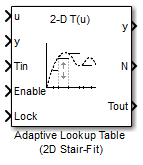Building Models Using Adaptive Lookup Table Blocks

Simulink® Design Optimization™ software provides blocks for modeling systems as adaptive lookup tables. You can use the adaptive lookup table blocks to create lookup tables from measured or simulated data. You build a model using the adaptive lookup table blocks, and then simulate the model to adapt the lookup table values to the time-varying I/O data. During simulation, the software uses the input data to locate the table values, and then uses the output data to recalculate the table values. The updated table values are stored in the adaptive lookup table block. For more information, see What are Adaptive Lookup Tables?.

The Adaptive Lookup Table library has the following blocks:

To access the Adaptive Lookup Tables library:

1. Open the Simulink Library Browser.

At the MATLAB® prompt, enter slLibraryBrowser.

2. Open the Simulink Design Optimization library.

In the Libraries pane, expand the Simulink Design Optimization node.

3. In the Simulink Design Optimization library tree, click Adaptive Lookup Tables.By default, the Adaptive Lookup Table blocks have two inputs and outputs. You can display additional inputs and outputs in a block by selecting the corresponding options in the Function Block Parameters dialog box. To learn more about the options, see the block reference pages.

Adaptive Lookup Table Block Showing Inputs and OutputsThe 2-D Adaptive Lookup Table block has the following inputs and outputs:

• u and y — Input and output data of the system being modeled, respectively.

For example, to model an engine's efficiency as a function of engine rpm and manifold pressure, specify u as the rpm, y as the pressure, and y as the efficiency signals.

• Tin — The initial table data.

• Enable — Signal to enable, disable, or reset the adaptation process.

• Lock — Signal to update only specified cells in the table.

• y — Value of the cell currently being adapted.

• N — Number of the cell currently being adapted.

• Tout — Values of the adapted table data.

A typical Simulink diagram using an adaptive lookup table block is shown in the next figure.In this figure, the Experiment Data block imports a set of experimental data into Simulink through MATLAB workspace variables. The initial table is specified in the block mask parameters. When the simulation runs, the initial table begins to adapt to new data inputs and the resulting table is copied to the block's output.